# Rescale MACD and RSI13

When placing MACD and RSI on the same window in MT5, they are automatically rescaled to fit on the same coordinate axis. I am trying to create an EA with these two indicators and need to points where they will intersect each other. However, I am unable to rescale the MACD to be on the same scale as the RSI in order to find the exact intersection points as would be found on the automatic rescaling of the software itself. I hope that makes sense...Moderator
164327

doriov :

When placing MACD and RSI on the same window in MT5, they are automatically rescaled to fit on the same coordinate axis. I am trying to create an EA with these two indicators and need to points where they will intersect each other. However, I am unable to rescale the MACD to be on the same scale as the RSI in order to find the exact intersection points as would be found on the automatic rescaling of the software itself. I hope that makes sense...

In the meantime, read the help (look carefully at the word "handle") :

iRSI

```int  iRSI(
string              symbol,            // symbol name
ENUM_TIMEFRAMES     period,            // period
int                 ma_period,         // averaging period
ENUM_APPLIED_PRICE  applied_price      // type of price or handle
);```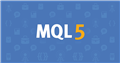Documentation on MQL5: Technical Indicators / iRSI
• www.mql5.com
//|                                                    Demo_iRSI.mq5 | //|                        Copyright 2011, MetaQuotes Software Corp. | //|                                             https://www.mql5.com | "The method of creation of the handle is set through the 'type' parameter (function type...Moderator
164327

Here's how we work with indicators manually:

• add the RSI indicator (ATTENTION: add from the Navigator window !!!) -> drag the RSI indicator from the Navigator window to the MACD window,
• in the "Parameters" tab, select "Previous Indicator's Data")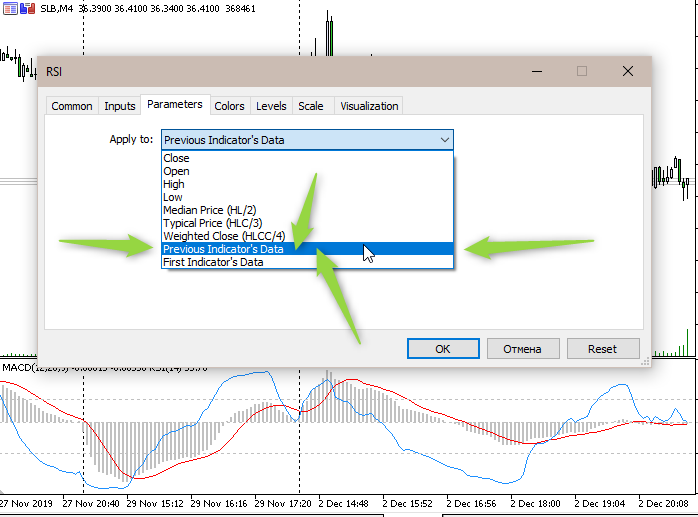And in the adviser, we act differently: we create the MACD handle, and when creating the RSI handle we write the MACD handle there:

```//+------------------------------------------------------------------+
//| Expert initialization function                                   |
//+------------------------------------------------------------------+
int OnInit()
{
//--- create handle of the indicator iMACD
handle_iMACD=iMACD(Symbol(),Period(),Inp_MACD_fast_ema_period,Inp_MACD_slow_ema_period,
Inp_MACD_signal_period,Inp_MACD_applied_price);
//--- if the handle is not created
if(handle_iMACD==INVALID_HANDLE)
{
//--- tell about the failure and output the error code
PrintFormat("Failed to create handle of the iMACD indicator for the symbol %s/%s, error code %d",
Symbol(),
EnumToString(Period()),
GetLastError());
//--- the indicator is stopped early
return(INIT_FAILED);
}
//--- create handle of the indicator iRSI
handle_iRSI=iRSI(Symbol(),Period(),Inp_RSI_ma_period,handle_iMACD);
//--- if the handle is not created
if(handle_iRSI==INVALID_HANDLE)
{
//--- tell about the failure and output the error code
PrintFormat("Failed to create handle of the iRSI indicator for the symbol %s/%s, error code %d",
Symbol(),
EnumToString(Period()),
GetLastError());
//--- the indicator is stopped early
return(INIT_FAILED);
}
//---
return(INIT_SUCCEEDED);
}```

Result (indicator Windows 3) :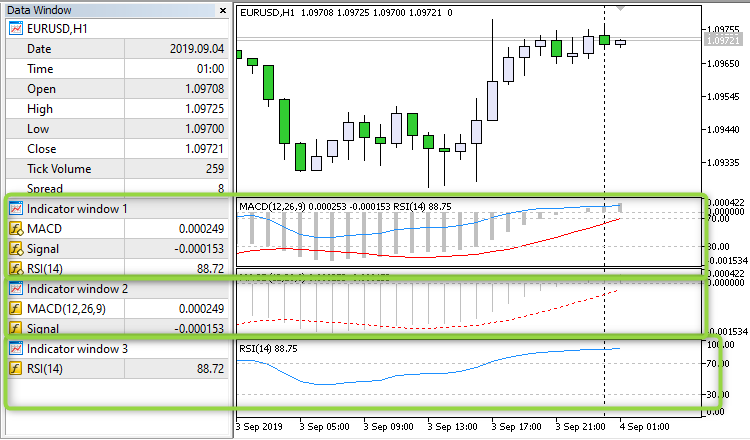Files:Moderator
164327

And now the main thing: the coefficient cannot be picked up - since this coefficient is floating, the coefficient changes all the time.

Look at an example: at first there seems to be an intersection of MACD and RSI, but if you move the chart, the intersection disappears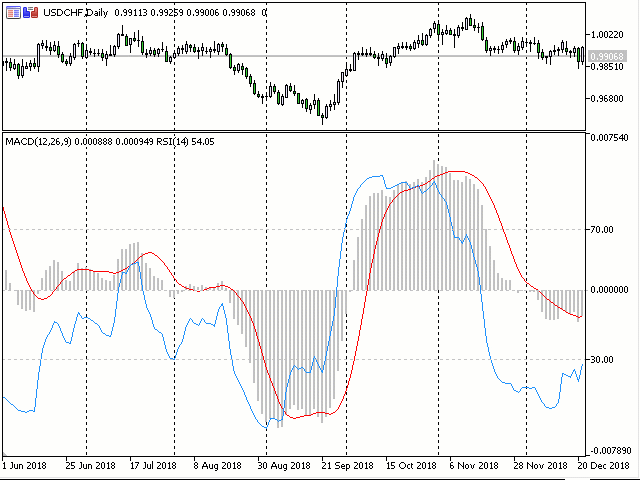212

The RSI is a bounded indicator, 0 to 100, while the MACD does not. There is therefore no point of comparison possible between the two since there is no common reference.

Try that bounded indicators between them: RSI, CCI, STOCK, WPR etc ...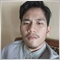329

stanislass:

The RSI is a bounded indicator, 0 to 100, while the MACD does not. There is therefore no point of comparison possible between the two since there is no common reference.

Try that bounded indicators between them: RSI, CCI, STOCK, WPR etc ...
I agree with you, need to provide additional conditions for MACD restriction points if you want to do it in conjunction with RSI.  It takes some research so that it can be done properly.329

And now the main thing: the coefficient cannot be picked up - since this coefficient is floating, the coefficient changes all the time.

Look at an example: at first there seems to be an intersection of MACD and RSI, but if you move the chart, the intersection disappears

in my opinion, will it be optimal if there is a limit for MACD transactions at a value of -300 to 300 and added to the direction of the MACD trend?  I think it will match the RSI from 0 to 100.Moderator
164327